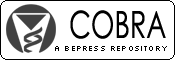## COBRA Preprint Series

#### Abstract

The reasoning behind uses of confidence intervals and p-values in scientific practice may be made coherent by modeling the inferring statistician or scientist as an idealized intelligent agent. With other things equal, such an agent regards a hypothesis coinciding with a confidence interval of a higher confidence level as more certain than a hypothesis coinciding with a confidence interval of a lower confidence level. The agent uses different methods of confidence intervals conditional on what information is available. The coherence requirement means all levels of certainty of hypotheses about the parameter agree with the same distribution of certainty over parameter space. The result is a unique and coherent fiducial distribution that encodes the post-data certainty levels of the agent.

While many coherent fiducial distributions coincide with confidence distributions or Bayesian posterior distributions, there is a general class of coherent fiducial distributions that equates the two-sided p-value with the probability that the null hypothesis is true. The use of that class leads to point estimators and interval estimators that can be derived neither from the dominant frequentist theory nor from Bayesian theories that rule out data-dependent priors. These simple estimators shrink toward the parameter value of the null hypothesis without relying on asymptotics or on prior distributions.

#### Disciplines

Statistical Methodology | Statistical Theory

COinS